Hello, Guest!
 Instructional Focus DocumentGeometry
 TITLE : Unit 06: Relationships of Circles, including Radian Measure and Equations of Circles SUGGESTED DURATION : 12 days

#### Unit Overview

Introduction
This unit bundles student expectations that address properties and attributes of circles, equations of circles, and angle and segment relationships within circles. Concepts are incorporated into both mathematical and real-world problem situations. According to the Texas Education Agency, mathematical process standards including application, tools and techniques, communication, representations, relationships, and justifications should be integrated (when applicable) with content knowledge and skills so that students are prepared to use mathematics in everyday life, society, and the workplace.

Prior to this Unit
In Grade 04, students investigated the properties and attributes of circles. In Grade 7, students defined pi, π, as the ratio of the circumference to the diameter of a circle.

During this Unit
Students define new circle vocabulary, including special segments and angles of circles, using diagrams and definitions. Students use patterns, diagrams, and a variety of tools to investigate circles, chords, secants, tangents, and their angle relationships, including central and inscribed angles. Students use patterns, diagrams, and a variety of tools to investigate circles, chords, secants, tangents, and their segment length relationships. Students apply theorems about combined circle angle/segment length relationships, including central and inscribed angles, in non-contextual problems. Students describe and develop the concept of radian measure of an angle as the ratio of the length of an arc intercepted by a central angle and the radius of the circle. Students convert between degree and radian measures. Students develop the equation of a circle, x² + y² = r², using the coordinate grid and the Pythagorean Theorem, given the radius, r, and center at the origin. Students determine the equation of a circle, (xh)² + (yk)² = r², given the radius and a center of (h, k). Students represent real-world situations using equations of circles.

Other considerations: Reference the Mathematics COVID-19 Gap Implementation Tool HS Geometry

After this Unit
In Unit 08, students will apply the proportional relationship between the measure of an arc length of a circle and the circumference of the circle and between the measure of the area of a sector of a circle and the area of the circle to solve problems. In subsequent courses in mathematics, these concepts will continue to be applied to problem situations involving circles.

This unit is supporting the development of Texas College Career Readiness Standards (TxCCRS): I. Numeric Reasoning B1, C1, C2; II. Algebraic Reasoning D1, D2; III. Geometric and Spatial Reasoning A1, A2, C1, D3; V. Statistical Reasoning A1, C2; VII. Problem Solving and Reasoning A1, A2, A3, A4, A5, B1, C1, C2, D1, D2; VIII. Communication and Representation A1, A2, A3, B1, B2, C1, C2, C3; IX. Connections A1, A2, B1, B2, B3.

Research
According to the National Council of Teachers of Mathematics (NCTM) in Focus in High School Mathematics: Reasoning and Sense Making (2009), the key elements of reasoning and sense making with geometry must include multiple representations of functions. In this unit, students gather data from geometric figures, and organize this information into tables, graphs or diagrams. This leads to the development of symbolic expressions and verbal descriptions. A variety of representations helps make relationships more understandable to more students than working with symbolic representations alone. These approaches serve as the basis for this unit on polygons and circle. At the conclusion of this unit, students are asked to create graphic organizers. TxCCRS cites many skills related to the communication and representation of mathematical ideas. The National Council of Teachers of Mathematics (2000) said that all students in grades 9 – 12 should explore relationships in two-dimensional geometric figures, make and test conjectures about two-dimensional geometric figures, and solve problems involving two-dimensional geometric figures. According to the National Council of Teachers of Mathematics (2012), using diagrams and constructions to interpret and communicate geometric relationships is essential in geometry. Using definitions of figures to characterize figures in terms of their properties is another essential in geometry. In geometry, the “proving process involves working with diagrams, variation and invariance, conjectures, and definitions.” (p. 92)

National Council of Teachers of Mathematics. (2000). Principles and standards for school mathematics. Reston, VA: National Council of Teachers of Mathematics, Inc.
National Council of Teachers of Mathematics. (2009). Focus in High School Mathematics: Reasoning and Sense Making. Reston, VA: National Council of Teachers of Mathematics, Inc.
National Council of Teachers of Mathematics. (2012). Developing essential understanding of Geometry for Teaching Mathematics in Grades 9 – 12. Reston, VA: National Council of Teachers of Mathematics, Inc.
Texas Education Agency & Texas Higher Education Coordinating Board. (2009). Texas college and career readiness standards. Retrieved from http://www.thecb.state.tx.us/index.cfm?objectid=E21AB9B0-2633-11E8-BC500050560100A9

 Geometric, spatial, and measurement reasoning are foundational to visualizing, analyzing, and applying relationships within and between scale, shapes, quantities, and spatial relations in everyday life. Why is developing geometric, spatial, and measurement reasoning essential? How does geometric, spatial, and measurement reasoning affect how one sees and works in the world? Logical reasoning can be used to make sense of claims, determine their validity, and construct and communicate arguments. Why is developing logical reasoning in mathematics important and how does this reasoning influence decision making in everyday life? What elements of logical reasoning influence the truth of a statement? How is logical reasoning used to uncover truths and/or make sense of, construct, and determine the validity of arguments and claims?
Unit Understandings
and Questions
Overarching Concepts
and Unit Concepts
Performance Assessment(s)
• Understanding and working with variance and invariance within geometry builds flexible algebraic and geometric reasoning and deepens understanding of intrinsic properties of geometric relationships.
• What invariant (unchanging) and variant (changing) relationships exist within circles?
• How does examining variance and invariance lead to new conjectures and theorems about circle relationships?
• Deductive reasoning can be used to determine the validity of a conditional statement and its related statements and conjectures about geometric relationships in order to support or refute mathematical claims through the process of proving.
• How is deductive reasoning used to understand, prove, and apply geometric conjectures about circles?
• angle measure relationships?
• special circle segment length relationships?
• combined measure relationships?
• Accurate representations, models, or diagrams within a geometric system allows for visualizing, illustrating, and analyzing geometric relationships to aid in making and validating conjectures about those geometric relationships and is central to geometric thinking.
• What types of problem situations represent circle relationships?
• How can representations and appropriate geometric language be used to effectively communicate and illustrate geometric relationships about circle relationships?
• What tools and processes can be used to investigate …
• circles?
• the relationships between arc angle measures and arc length measures?
• special segments of circles?
• special angles of circles?
• How can constructions be used to make and validate conjectures about geometric relationships in circles?
• relationships in angles of circles?
• relationships in special circle segments?
• Attributes and quantifiable measures of geometric figures can be generalized to describe, determine, and represent algebraic and geometric relationships and be applied to solve problem
• How can understanding circle relationships be applied when solving problem situations?
• How can measurable attributes related to …
• angle measures, including central and inscribed angles
• arc lengths and arc angles
• special circle segments lengths, including radii, chords, tangents, and secants
… be distinguished and described in order to generalize geometric relationships of circles?
• What processes can be used to determine the …
• angle measure of a circle?
• length of a segment of a circle?
• combined angle measures of a circle?
• combined segment lengths of a circle?
• Circles
• Circles
• Theorems
• Circle relationships
• Measure relationships
• Geometric Relationships
• Congruence
• Equidistance
• Equivalence
• Perpendicularity
• Formulas
• Geometric Representations
• Two-dimensional figures
• Triangle Relationships
• Right triangles
• Logical Arguments and Constructions
• Constructions
• Deductive Reasoning
• Definitions
• Conjectures
• Theorems
• Geometric Relationships
• Angle relationships
• Geometric Representations
• Angles
• Segments
• Circles
• Patterns, Operations, and Properties
• Associated Mathematical Processes
• Problem Solving Model
• Tools and Techniques
• Communication
• Representations
• Relationships
• Justification
 Assessment information provided within the TEKS Resource System are examples that may, or may not, be used by your child’s teacher. In accordance with section 26.006 (2) of the Texas Education Code, "A parent is entitled to review each test administered to the parent’s child after the test is administered." For more information regarding assessments administered to your child, please visit with your child’s teacher.

 Geometric, spatial, and measurement reasoning are foundational to visualizing, analyzing, and applying relationships within and between scale, shapes, quantities, and spatial relations in everyday life. Why is developing geometric, spatial, and measurement reasoning essential? How does geometric, spatial, and measurement reasoning affect how one sees and works in the world? Logical reasoning can be used to make sense of claims, determine their validity, and construct and communicate arguments. Why is developing logical reasoning in mathematics important and how does this reasoning influence decision making in everyday life? What elements of logical reasoning influence the truth of a statement? How is logical reasoning used to uncover truths and/or make sense of, construct, and determine the validity of arguments and claims?
Unit Understandings
and Questions
Overarching Concepts
and Unit Concepts
Performance Assessment(s)
• Understanding and working with variance and invariance within geometry builds flexible algebraic and geometric reasoning and deepens understanding of intrinsic properties of geometric relationships.
• What invariant (unchanging) and variant (changing) relationships exist within circles?
• How does examining variance and invariance lead to new conjectures about circle relationships?
• Deductive reasoning can be used to determine the validity of a conditional statement and its related statements and conjectures about geometric relationships in order to support or refute mathematical claims through the process of proving.
• angle measure relationships?
• segment length relationships?
• Accurate representations, models, or diagrams within a geometric system allows for visualizing, illustrating, and analyzing geometric relationships to aid in making and validating conjectures about those geometric relationships and is central to geometric thinking.
• What types of problem situations represent circle relationships?
• How can representations and appropriate geometric language be used to effectively communicate and illustrate geometric relationships about circle relationships?
• What tools and processes can be used to investigate …
• circles?
• arcs and radii of circles?
• central angles of circles?
• degree and radian measure of angles of circles?
• equations of circles?
• How can the coordinate plane be used to make and validate conjectures about geometric relationships in circles?
• relationships in angles of circles?
• relationships in special circle segments?
• the relationship of the equation of a circle and the Pythagorean Theorem?
• Attributes and quantifiable measures of geometric figures can be generalized to describe, determine, and represent algebraic and geometric relationships and be applied to solve problem situations.
• How can understanding circle relationships be applied when solving problem situations?
• How can measurable attributes related to …
• distance
• special segments of circles, including arcs and radii
• Pythagorean Theorem
• degree and radian measure of angles of circles
… be distinguished and described in order to generalize geometric relationships of circles?
• What processes can be used to determine the …
• degree or radian measure of an angle of a circle?
• equation of a circle?
• Coordinate and Transformational Geometry; Circles
• Circles
• Circle relationships
• Measure relationships
• Equation of a circle
• Geometric Relationships
• Equidistance
• Equivalence
• Proportionality
• Formulas
• Geometric Representations
• Two-dimensional figures
• Two-Dimensional Coordinate Systems
• Distance
• Logical Arguments and Constructions
• Deductive Reasoning
• Definitions
• Conjectures
• Geometric Relationships
• Angle relationships
• Geometric Representations
• Angles
• Segments
• Circles
• Patterns, Operations, and Properties
• Associated Mathematical Processes
• Application
• Problem Solving Model
• Tools and Techniques
• Communication
• Representations
• Relationships
• Justification
 Assessment information provided within the TEKS Resource System are examples that may, or may not, be used by your child’s teacher. In accordance with section 26.006 (2) of the Texas Education Code, "A parent is entitled to review each test administered to the parent’s child after the test is administered." For more information regarding assessments administered to your child, please visit with your child’s teacher.

#### MISCONCEPTIONS / UNDERDEVELOPED CONCEPTS

Misconceptions:

• Some students may think angles formed by intersecting chords are central angles even if the intersection is not at the center of the circle.
• Some students may think that a major arc can be named using only two points on the circle rather than using three points on the circle.
• Some students may think pi,, is just a number rather than a relationship between the circumference and radius of a circle.

Underdeveloped Concepts:

• Some students may think thatequals 180° instead ofradians equals 180° or approximately 3.14 radians equals 180°.
• Some students may think that if the vertex of an angle is on the circle the angle measure is the same as the measure of the intercepted arc rather than one-half the measure of the intercepted arc.
• Some students may determine the measure of an exterior angle created by the intersection of secants and/or tangents to be one-half the sum of the measures of the intercepted arcs rather than one-half the difference of the intercepted arcs.
• Some students may not recognize or useas a variable.
• Some students may not realize that leavingin an equation or formula represents an exact value.
• Some students may always use (0, 0) as the (h, k) when writing the equation of a circle.
• Some students may fail to take the square root of the r2 portion of the equation of a circle when determining the radius of the circle.

#### Unit Vocabulary

• Center of a circle – point equidistant from all points on the circle
• Central angle – angle whose vertex is the center of the circle and whose sides are radii of the circle
• Chord of a circle – line segment that joins two points on the circle
• Circle – set of all points equidistant from a given point called the center of the circle
• Conjecture – statement believed to be true but not yet proven
• Diameter – a line segment whose endpoints are on the circle and passes through the center of the circle
• Inscribed angle – angle whose vertex is on the circle and whose sides are chords of the circle
• Radian measure – ratio of the length of an arc intercepted by a central angle and the radius of the circle
• Radius – line segment drawn from the center of a circle to any point on the circle and is half the length of the diameter of the circle
• Secant to a circle – line, ray, or line segment that intersects the circle in exactly two points
• Tangent to a circle – line, ray, or line segment perpendicular to the radius and intersecting the circle at exactly one point, the point of tangency

Related Vocabulary:

 Arc Circumference Distance formula Equation of a circle Intercepted arc Major arc Minor arc Perpendicular bisector Point of tangency Semicircle Tangent segment
Unit Assessment Items System Resources Other Resources

Show this message:

Unit Assessment Items that have been published by your district may be accessed through Search All Components in the District Resources tab. Assessment items may also be found using the Assessment Center if your district has granted access to that tool.

System Resources may be accessed through Search All Components in the District Resources Tab.

Texas Higher Education Coordinating Board – Texas College and Career Readiness Standards

Texas Education Agency – Mathematics Curriculum

Texas Education Agency – STAAR Mathematics Resources

Texas Education Agency Texas Gateway – Revised Mathematics TEKS: Vertical Alignment Charts

Texas Education Agency Texas Gateway – Mathematics TEKS: Supporting Information

Texas Education Agency Texas Gateway – Interactive Mathematics Glossary

Texas Education Agency Texas Gateway – Resources Aligned to Geometry Mathematics TEKS

Texas Instruments – Graphing Calculator Tutorials

TAUGHT DIRECTLY TEKS

TEKS intended to be explicitly taught in this unit.

TEKS/SE Legend:

• Knowledge and Skills Statements (TEKS) identified by TEA are in italicized, bolded, black text.
• Student Expectations (TEKS) identified by TEA are in bolded, black text.
• Portions of the Student Expectations (TEKS) that are not included in this unit but are taught in previous or future units are indicated by a strike-through.

Specificity Legend:

• Supporting information / clarifications (specificity) written by TEKS Resource System are in blue text.
• Unit-specific clarifications are in italicized, blue text.
• Information from Texas Education Agency (TEA), Texas College and Career Readiness Standards (TxCCRS), Texas Response to Curriculum Focal Points (TxRCFP) is labeled.
• A Partial Specificity label indicates that a portion of the specificity not aligned to this unit has been removed.
TEKS# SE# TEKS SPECIFICITY
G.1 Mathematical process standards. The student uses mathematical processes to acquire and demonstrate mathematical understanding. The student is expected to:
G.1A Apply mathematics to problems arising in everyday life, society, and the workplace.

Apply

MATHEMATICS TO PROBLEMS ARISING IN EVERYDAY LIFE, SOCIETY, AND THE WORKPLACE

Including, but not limited to:

• Mathematical problem situations within and between disciplines
• Everyday life
• Society
• Workplace

Note(s):

• The mathematical process standards may be applied to all content standards as appropriate.
• TxCCRS:
• VII.D. Problem Solving and Reasoning – Real-world problem solving
• VII.D.1. Interpret results of the mathematical problem in terms of the original real-world situation.
• IX.A. Connections – Connections among the strands of mathematics
• IX.A.1. Connect and use multiple key concepts of mathematics in situations and problems.
• IX.A.2. Connect mathematics to the study of other disciplines.
• IX.B. Connections – Connections of mathematics to nature, real-world situations, and everyday life
• IX.B.1. Use multiple representations to demonstrate links between mathematical and real-world situations.
• IX.B.2. Understand and use appropriate mathematical models in the natural, physical, and social sciences.
• IX.B.3. Know and understand the use of mathematics in a variety of careers and professions.
G.1B Use a problem-solving model that incorporates analyzing given information, formulating a plan or strategy, determining a solution, justifying the solution, and evaluating the problem-solving process and the reasonableness of the solution.

Use

A PROBLEM-SOLVING MODEL THAT INCORPORATES ANALYZING GIVEN INFORMATION, FORMULATING A PLAN OR STRATEGY, DETERMINING A SOLUTION, JUSTIFYING THE SOLUTION, AND EVALUATING THE PROBLEM-SOLVING PROCESS AND THE REASONABLENESS OF THE SOLUTION

Including, but not limited to:

• Problem-solving model
• Analyze given information
• Formulate a plan or strategy
• Determine a solution
• Justify the solution
• Evaluate the problem-solving process and the reasonableness of the solution

Note(s):

• The mathematical process standards may be applied to all content standards as appropriate.
• TxCCRS:
• I.B. Numeric Reasoning – Number sense and number concepts
• I.B.1. Use estimation to check for errors and reasonableness of solutions.
• V.A. Statistical Reasoning – Design a study
• V.A.1. Formulate a statistical question, plan an investigation, and collect data.
• VII.A. Problem Solving and Reasoning – Mathematical problem solving
• VII.A.1. Analyze given information.
• VII.A.2. Formulate a plan or strategy.
• VII.A.3. Determine a solution.
• VII.A.4. Justify the solution.
• VII.A.5. Evaluate the problem-solving process.
• VII.D. Problem Solving and Reasoning – Real-world problem solving
• VII.D.2. Evaluate the problem-solving process.
G.1C Select tools, including real objects, manipulatives, paper and pencil, and technology as appropriate, and techniques, including mental math, estimation, and number sense as appropriate, to solve problems.

Select

TOOLS, INCLUDING REAL OBJECTS, MANIPULATIVES, PAPER AND PENCIL, AND TECHNOLOGY AS APPROPRIATE, AND TECHNIQUES, INCLUDING MENTAL MATH, ESTIMATION, AND NUMBER SENSE AS APPROPRIATE, TO SOLVE PROBLEMS

Including, but not limited to:

• Appropriate selection of tool(s) and techniques to apply in order to solve problems
• Tools
• Real objects
• Manipulatives
• Paper and pencil
• Technology
• Techniques
• Mental math
• Estimation
• Number sense

Note(s):

• The mathematical process standards may be applied to all content standards as appropriate.
• TxCCRS:
• I.B. Numeric Reasoning – Number sense and number concepts
• I.B.1. Use estimation to check for errors and reasonableness of solutions.
• V.C. Statistical Reasoning – Analyze, interpret, and draw conclusions from data
• V.C.2. Analyze relationships between paired data using spreadsheets, graphing calculators, or statistical software.
G.1D Communicate mathematical ideas, reasoning, and their implications using multiple representations, including symbols, diagrams, graphs, and language as appropriate.

Communicate

MATHEMATICAL IDEAS, REASONING, AND THEIR IMPLICATIONS USING MULTIPLE REPRESENTATIONS, INCLUDING SYMBOLS, DIAGRAMS, GRAPHS, AND LANGUAGE AS APPROPRIATE

Including, but not limited to:

• Mathematical ideas, reasoning, and their implications
• Multiple representations, as appropriate
• Symbols
• Diagrams
• Graphs
• Language

Note(s):

• The mathematical process standards may be applied to all content standards as appropriate.
• TxCCRS:
• II.D. Algebraic Reasoning – Representing relationships
• II.D.1. Interpret multiple representations of equations, inequalities, and relationships.
• II.D.2. Convert among multiple representations of equations, inequalities, and relationships.
• VIII.A. Communication and Representation – Language, terms, and symbols of mathematics
• VIII.A.1. Use mathematical symbols, terminology, and notation to represent given and unknown information in a problem.
• VIII.A.2. Use mathematical language to represent and communicate the mathematical concepts in a problem.
• VIII.A.3. Use mathematical language for reasoning, problem solving, making connections, and generalizing.
• VIII.B. Communication and Representation – Interpretation of mathematical work
• VIII.B.1. Model and interpret mathematical ideas and concepts using multiple representations.
• VIII.B.2. Summarize and interpret mathematical information provided orally, visually, or in written form within the given context.
• VIII.C. Communication and Representation – Presentation and representation of mathematical work
• VIII.C.1. Communicate mathematical ideas, reasoning, and their implications using symbols, diagrams, models, graphs, and words.
• VIII.C.2. Create and use representations to organize, record, and communicate mathematical ideas.
• VIII.C.3. Explain, display, or justify mathematical ideas and arguments using precise mathematical language in written or oral communications.
• IX.B. Connections – Connections of mathematics to nature, real-world situations, and everyday life
• IX.B.1. Use multiple representations to demonstrate links between mathematical and real-world situations.
G.1E Create and use representations to organize, record, and communicate mathematical ideas.

Create, Use

REPRESENTATIONS TO ORGANIZE, RECORD, AND COMMUNICATE MATHEMATICAL IDEAS

Including, but not limited to:

• Representations of mathematical ideas
• Organize
• Record
• Communicate
• Evaluation of the effectiveness of representations to ensure clarity of mathematical ideas being communicated
• Appropriate mathematical vocabulary and phrasing when communicating mathematical ideas

Note(s):

• The mathematical process standards may be applied to all content standards as appropriate.
• TxCCRS:
• VIII.B. Communication and Representation – Interpretation of mathematical work
• VIII.B.1. Model and interpret mathematical ideas and concepts using multiple representations.
• VIII.B.2. Summarize and interpret mathematical information provided orally, visually, or in written form within the given context.
• VIII.C. Communication and Representation – Presentation and representation of mathematical work
• VIII.C.1. Communicate mathematical ideas, reasoning, and their implications using symbols, diagrams, models, graphs, and words.
• VIII.C.2. Create and use representations to organize, record, and communicate mathematical ideas.
G.1F Analyze mathematical relationships to connect and communicate mathematical ideas.

Analyze

MATHEMATICAL RELATIONSHIPS TO CONNECT AND COMMUNICATE MATHEMATICAL IDEAS

Including, but not limited to:

• Mathematical relationships
• Connect and communicate mathematical ideas
• Conjectures and generalizations from sets of examples and non-examples, patterns, etc.
• Current knowledge to new learning

Note(s):

• The mathematical process standards may be applied to all content standards as appropriate.
• TxCCRS:
• VII.A. Problem Solving and Reasoning – Mathematical problem solving
• VII.A.1. Analyze given information.
• VIII.A. Communication and Representation – Language, terms, and symbols of mathematics
• VIII.A.1. Use mathematical symbols, terminology, and notation to represent given and unknown information in a problem.
• VIII.A.2. Use mathematical language to represent and communicate the mathematical concepts in a problem.
• VIII.A.3. Use mathematical language for reasoning, problem solving, making connections, and generalizing.
•  VIII.B. Communication and Representation – Interpretation of mathematical work
• VIII.B.1. Model and interpret mathematical ideas and concepts using multiple representations.
• VIII.C. Communication and Representation – Presentation and representation of mathematical work
• VIII.C.1. Communicate mathematical ideas, reasoning, and their implications using symbols, diagrams, models, graphs, and words.
• VIII.C.2. Create and use representations to organize, record, and communicate mathematical ideas.
• VIII.C.3. Explain, display, or justify mathematical ideas and arguments using precise mathematical language in written or oral communications.
• IX.A. Connections – Connections among the strands of mathematics
• IX.A.1. Connect and use multiple key concepts of mathematics in situations and problems.
• IX.A.2. Connect mathematics to the study of other disciplines.
G.1G Display, explain, and justify mathematical ideas and arguments using precise mathematical language in written or oral communication.

Display, Explain, Justify

MATHEMATICAL IDEAS AND ARGUMENTS USING PRECISE MATHEMATICAL LANGUAGE IN WRITTEN OR ORAL COMMUNICATION

Including, but not limited to:

• Mathematical ideas and arguments
• Validation of conclusions
• Displays to make work visible to others
• Diagrams, visual aids, written work, etc.
• Explanations and justifications
• Precise mathematical language in written or oral communication

Note(s):

• The mathematical process standards may be applied to all content standards as appropriate.
• TxCCRS:
• VII.A. Problem Solving and Reasoning – Mathematical problem solving
• VII.A.4. Justify the solution.
• VII.B. Problem Solving and Reasoning – Proportional reasoning
• VII.B.1. Use proportional reasoning to solve problems that require fractions, ratios, percentages, decimals, and proportions in a variety of contexts using multiple representations.
• VII.C. Problem Solving and Reasoning – Logical reasoning
• VII.C.1. Develop and evaluate convincing arguments.
• VIII.A. Communication and Representation – Language, terms, and symbols of mathematics
• VIII.A.3. Use mathematical language for reasoning, problem solving, making connections, and generalizing.
• VIII.B. Communication and Representation – Interpretation of mathematical work
• VIII.B.1. Model and interpret mathematical ideas and concepts using multiple representations.
• VIII.B.2. Summarize and interpret mathematical information provided orally, visually, or in written form within the given context.
• VIII.C. Communication and Representation – Presentation and representation of mathematical work
• VIII. C.3. Explain, display, or justify mathematical ideas and arguments using precise mathematical language in written or oral communications.
G.2 Coordinate and transformational geometry. The student uses the process skills to understand the connections between algebra and geometry and uses the one- and two-dimensional coordinate systems to verify geometric conjectures. The student is expected to:
G.2B

Derive and use the distance, slope, and midpoint formulas to verify geometric relationships, including congruence of segments and parallelism or perpendicularity of pairs of lines.

Use

THE DISTANCE FORMULA TO VERIFY GEOMETRIC RELATIONSHIPS

Including, but not limited to:

• Distance formula: d =• Equation of a line
• Slope-intercept form, y = mx + b
• Point-slope form, yy1 = m(xx1)
• Standard form, Ax + By = C
• Relationships of special segments and points in circles
• Center of circle
• Circle – set of all points equidistant from a given point called the center of the circle
• Center of a circle – point equidistant from all points on the circle
• Chord of a circle
• Chord of a circle – line segment that joins two points on the circle
• Diameter and radius of a circle
• Diameter – a line segment whose endpoints are on the circle and passes through the center of the circle
• Radius – line segment drawn from the center of a circle to any point on the circle and is half the length of the diamter of the circle
• Tangent to a circle
• Tangent to a circle – line, ray, or line segment perpendicular to the radius and intersecting the circle at exactly one point, the point of tangency

Note(s):

• Grade 8 introduced and applied the Pythagorean Theorem to determine the distance between two points on a coordinate plane.
• Grade 8 introduced slope asor.
• Algebra I addressed determining equations of lines using point-slope form, slope intercept form, and standard form.
• Algebra I wrote equations of lines that contain a given point and are parallel or perpendicular to a given line.
• Various mathematical process standards will be applied to this student expectation as appropriate.
• TxCCRS
• III.C. Geometric and Spatial Reasoning – Connections between geometry and other mathematical content strands
• III.C.1. Make connections between geometry and algebraic equations.
• VII.B. Problem Solving and Reasoning – Proportional reasoning
• VII.B.1. Use proportional reasoning to solve problems that require fractions, ratios, percentages, decimals, and proportions in a variety of contexts using multiple representations.
• IX.A. Connections – Connections among the strands of mathematics
• IX.A.1. Connect and use multiple key concepts of mathematics in situations and problems.
G.5 Logical argument and constructions. The student uses constructions to validate conjectures about geometric figures. The student is expected to:
G.5A

Investigate patterns to make conjectures about geometric relationships, including angles formed by parallel lines cut by a transversal, criteria required for triangle congruence, special segments of triangles, diagonals of quadrilaterals, interior and exterior angles of polygons, and special segments and angles of circles choosing from a variety of tools.

Investigate

PATTERNS TO MAKE CONJECTURES ABOUT GEOMETRIC RELATIONSHIPS, INCLUDING SPECIAL SEGMENTS AND ANGLES OF CIRCLES CHOOSING FROM A VARIETY OF TOOLS

Including, but not limited to:

• Conjecture – statement believed to be true but not yet proven
• Investigations should include good sample design, valid conjecture, and inductive/deductive reasoning.
• Patterns include numeric and geometric properties.
• Utilization of a variety of tools in the investigations (e.g., compass and straightedge, paper folding, manipulatives, dynamic geometry software, technology)
• Special segments and angles of circles
• Central angle – angle whose vertex is the center of the circle and whose sides are radii of the circle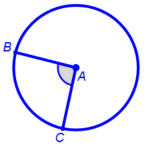• Measure of a central angle is equal to the measure of the intercepted arc.
• Inscribed angle – angle whose vertex is on the circle and whose sides are chords of the circle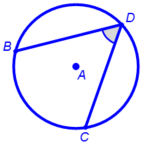• Measure of an inscribed angle is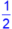the measure of the intercepted arc.
• Inscribed angle with semicircle intercepted arc is a right angle.
• Measure of an inscribed angle isthe measure of the central angle that shares the same intercepted arc.
• Chord of a circle – line segment that joins two points on the circle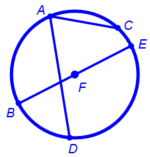• Two chords are congruent if and only if their corresponding intercepting arcs are congruent.
• Diameter of a circle bisects a chord if and only if the diameter and chord are perpendicular.
• Products of the lengths of the segments of intersecting chords are equal.
• Vertical angles formed by intersecting chords are equal in measure.
• Adjacent angles formed by intersecting chords are supplementary.
• Measure of the angle formed by intersecting chords isthe sum of the measures of the intercepted arcs.
• Secant to a circle – line, ray, or line segment that intersects the circle in exactly two points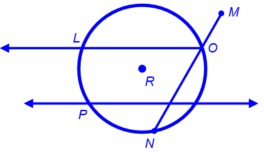• Secant-Secant
• Product of the length of the secant segment and its external segment is equal to the product of the other secant segment and its external segment from the same point outside the circle.
• Measure of the angle formed by two secants intersecting at a point outside the circle isthe difference of the measures of the intercepted arcs.
• Tangent to a circle – line, ray, or line segment perpendicular to the radius and intersecting the circle in exactly one point, the point of tangency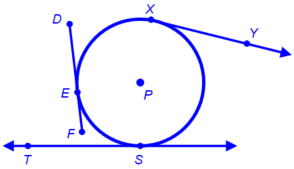• Tangent-Tangent
• Intersecting tangent segments are equal in length from the same point outside the circle.
• Measure of angle formed by the intersection of two tangents at a point outside the circle isthe difference of the measures of the intercepted arcs.
• Tangent line is perpendicular to a radius of the circle at the point of tangency.
• Combinations of chords, secants, and tangents
• Chord-Tangent
• Measure of the angle formed by the intersection of a tangent and chord isthe measure of the intercepted arc.
• Secant-Tangent
• Product of the length of a secant segment and its external part is equal to the product of the square of the length of a tangent segment intersecting the secant segment at a point outside the circle.
• Measure of the angle formed by a secant and a tangent intersecting at the same point outside the circle isthe difference of the measures of the intercepted arcs.

Note(s):

• Previous grade levels investigated attributes of geometric figures.
• Grade 8 used informal arguments to establish facts about the angle sum and exterior angle of triangles, the angles created when parallel lines are cut by a transversal, and the Angle-Angle criterion for similarity of triangles.
• Geometry introduces analyzing patterns in geometric relationships and making conjectures about geometric relationships which may or may not be represented using algebraic expressions.
• Various mathematical process standards will be applied to this student expectation as appropriate.
• TxCCRS
• III.A. Geometric and Spatial Reasoning – Figures and their properties
• III.A.2. Form and validate conjectures about one-, two-, and three-dimensional figures and their properties.
• III.D. Geometric and Spatial Reasoning – Measurements involving geometry and algebra
• III.D.3. Determine indirect measurements of geometric figures using a variety of methods.
• VII.A. Problem Solving and Reasoning – Mathematical problem solving
• VII.A.1. Analyze given information.
• VII.B. Problem Solving and Reasoning – Proportional reasoning
• VII.B.1. Use proportional reasoning to solve problems that require fractions, ratios, percentages, decimals, and proportions in a variety of contexts using multiple representations.
• VII.C. Problem Solving and Reasoning – Logical reasoning
• VII.C.1. Develop and evaluate convincing arguments.
• VII.C.2. Understand attributes and relationships with inductive and deductive reasoning.
G.12 Circles. The student uses the process skills to understand geometric relationships and apply theorems and equations about circles. The student is expected to:
G.12A Apply theorems about circles, including relationships among angles, radii, chords, tangents, and secants, to solve non-contextual problems.

Apply

THEOREMS ABOUT CIRCLES, INCLUDING RELATIONSHIPS AMONG ANGLES, RADII, CHORDS, TANGENTS, AND SECANTS TO SOLVE NON-CONTEXTUAL PROBLEMS

Including, but not limited to:

• Geometric relationships among angles, radii, chords, tangents, and secants
• Measure of the arc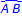is denoted as m.
• Central angle – angle whose vertex is the center of the circle and whose sides are radii of the circle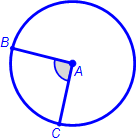• Measure of a central angle is equal to the measure of the intercepted arc.
• Inscribed angle – angle whose vertex is on the circle and whose sides are chords of the circle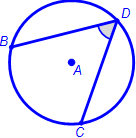• Measure of an inscribed angle isthe measure of the intercepted arc.
• Inscribed angle with semicircle intercepted arc is a right angle.
• Measure of an inscribed angle isthe measure of the central angle that shares the same intercepted arc.
• Radius of a circle is perpendicular to a tangent line at the point of tangency.
• Chord of a circle – line segment that joins two points on the circle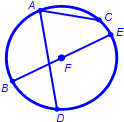• Two chords are congruent if and only if their corresponding intercepting arcs are congruent.
• Diameter of a circle bisects a chord if and only if the diameter and chord are perpendicular.
• Products of the lengths of the segments of intersecting chords are equal.
• Vertical angles formed by intersecting chords are equal in measure.
• Adjacent angles formed by intersecting chords are supplementary.
• Measure of an angle formed by intersecting chords isthe sum of the measures of the intercepted arcs.
• Tangent to a circle – line, ray, or line segment perpendicular to the radius and intersecting the circle in exactly one point, the point of tangency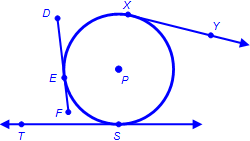• Intersecting tangent segments are equal in length from the same point outside the circle.
• Measure of angle formed by the intersection of two tangents outside the circle isthe difference of the measures of the intercepted arcs.
• Secant to a circle – line, ray, or line segment that intersects the circle in exactly two points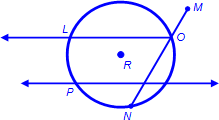• Product of the length of the secant segment and its external segment is equal to the product of the other secant segment and its external segment from the same point outside the circle.
• Measure of angle formed by two secants intersecting at a point outside the circle isthe difference of the measures of the intercepted arcs.
• Combinations of chords, secants, and tangents
• Chord-Tangent
• Measure of the angle formed by the intersection of a tangent and chord isthe measure of the intercepted arc.
• Secant-Tangent
• Product of the length of a secant segment and its external part is equal to the product of the square of the length of a tangent segment intersecting the secant segment at a point outside the circle.
• Measure of the angle formed by a secant and a tangent intersecting at the same point outside the circle isthe difference of the measures of the intercepted arcs.
• Applications to non-contextual mathematical problem situations
• Use of appropriate units of measure

Note(s):

• Previous grade levels explored characteristics of circles.
• Various mathematical process standards will be applied to this student expectation as appropriate.
• TxCCRS
• III.A. Geometric and Spatial Reasoning – Figures and their properties
• III.A.2. Form and validate conjectures about one-, two-, and three-dimensional figures and their properties.
• III.C. Geometric and Spatial Reasoning – Connections between geometry and other mathematical content strands
• III.C.1. Make connections between geometry and algebraic equations.
• VII.A. Problem Solving and Reasoning – Mathematical problem solving
• VII.A.3. Determine a solution.
• VII.B. Problem Solving and Reasoning – Proportional reasoning
• VII.B.1. Use proportional reasoning to solve problems that require fractions, ratios, percentages, decimals, and proportions in a variety of contexts using multiple representations.
• VII.C. Problem Solving and Reasoning – Logical reasoning
• VII.C.1. Develop and evaluate convincing arguments.
• VII.C.2. Understand attributes and relationships with inductive and deductive reasoning.
G.12D Describe radian measure of an angle as the ratio of the length of an arc intercepted by a central angle and the radius of the circle.

Describe

RADIAN MEASURE OF AN ANGLE AS THE RATIO OF THE LENGTH OF AN ARC INTERCEPTED BY A CENTRAL ANGLE AND THE RADIUS OF THE CIRCLE

Including, but not limited to:

• Radian measure – ratio of the length of an arc intercepted by a central angle and the radius of the circle
• Comparison of radian measure of a circle and degree measure of a circle
• Generalization of the common conversion factors between degree and radian measure
• 180° = π
• Conversions of degrees into radians and radians into degree measures (values in radians can be left in terms of π)
• Radian measure can be described as θ =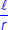, where θ is the radian measure of the central angle, ℓ is the length of the arc intercepted by the central angle, and r is the length of the radius of the circle

Note(s):

• Geometry lays the foundation for development of radian measurement in Precalculus.
• Various mathematical process standards will be applied to this student expectation as appropriate.
• TxCCRS
• I.C. Numeric Reasoning – Systems of measurement
• I.C.1. Select or use the appropriate type of method, unit, and tool for the attribute being measured.
• I.C.2. Convert units within and between systems of measurement.
• III.A. Geometric and Spatial Reasoning – Figures and their properties
• III.A.2. Form and validate conjectures about one-, two-, and three-dimensional figures and their properties.
• III.C. Geometric and Spatial Reasoning – Connections between geometry and other mathematical content strands
• III.C.1. Make connections between geometry and algebraic equations.
• VII.A. Problem Solving and Reasoning – Mathematical problem solving
• VII.A.1. Analyze given information.
• VII.B. Problem Solving and Reasoning – Proportional reasoning
• VII.B.1. Use proportional reasoning to solve problems that require fractions, ratios, percentages, decimals, and proportions in a variety of contexts using multiple representations.
• VII.C. Problem Solving and Reasoning – Logical reasoning
• VII.C.2. Understand attributes and relationships with inductive and deductive reasoning.
• VIII.A. Communication and Representation – Language, terms, and symbols of mathematics
• VIII.A.2. Use mathematical language to represent and communicate the mathematical concepts in a problem.
• VIII.C. Communication and Representation – Presentation and representation of mathematical work
• VIII.C.1. Communicate mathematical ideas, reasoning, and their implications using symbols, diagrams, models, graphs, and words.
• VIII.C.3. Explain, display, or justify mathematical ideas and arguments using precise mathematical language in written or oral communications.
G.12E Show that the equation of a circle with center at the origin and radius r is x2 + y2 = r2 and determine the equation for the graph of a circle with radius r and center (h, k), (x - h)2 + (y - k)2 =r2.

Show

THAT THE EQUATION OF A CIRCLE WITH CENTER AT THE ORIGIN AND RADIUS r: x2 + y2 = r2

Including, but not limited to:

• Derivation of equation of a circle using the distance formula

Determine

THE EQUATION FOR THE GRAPH OF A CIRCLE WITH RADIUS r AND CENTER (h, k), (x – h)2 + (yk)2 = r2

Including, but not limited to:

• General equation for a circle with center (h, k) and radius of length r: (x h)2 + (yk)2 = r2
• Graphs of circles from their equations
• Equations for circles given their graphs

Note(s):

• Geometry further develops the foundation of conic sections measurement in Precalculus.
• Various mathematical process standards will be applied to this student expectation as appropriate.
• TxCCRS
• III.A. Geometric and Spatial Reasoning – Figures and their properties
• III.A.1. Recognize characteristics and dimensional changes of two- and three-dimensional figures.
• III.A.2. Form and validate conjectures about one-, two-, and three-dimensional figures and their properties.
• III.C. Geometric and Spatial Reasoning – Connections between geometry and other mathematical content strands
• III.C.1. Make connections between geometry and algebraic equations.
• VII.A. Problem Solving and Reasoning – Mathematical problem solving
• VII.A.1. Analyze given information.
• VII.B. Problem Solving and Reasoning – Proportional reasoning
• VII.B.1. Use proportional reasoning to solve problems that require fractions, ratios, percentages, decimals, and proportions in a variety of contexts using multiple representations.
• VII.C. Problem Solving and Reasoning – Logical reasoning
• VII.C.2. Understand attributes and relationships with inductive and deductive reasoning.
• IX.A. Connections – Connections among the strands of mathematics
• IX.A.1. Connect and use multiple key concepts of mathematics in situations and problems.